x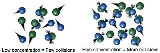Collision theoryEncyclopedia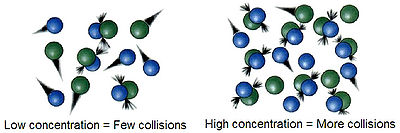Collision theory is a theory proposed by Max Trautz
Max Trautz
Max Trautz was a German chemist. He was very productive with over 190 scientific publications especially in the field of chemical kinetics...

and William Lewis
William Lewis
William Lewis was involved in English Association Football in various capacities in the early 20th century. He was first a referee, then a director and later manager of Brentford between 1900 and 1903. He became the first club secretary of Chelsea Football Club when it was founded in 1905, with his...

in 1916 and 1918, that qualitatively explains how chemical reactions occur and why reaction rate
Reaction rate
The reaction rate or speed of reaction for a reactant or product in a particular reaction is intuitively defined as how fast or slow a reaction takes place...

s differ for different reactions. For a reaction to occur the reactant particles must collide. Only a certain fraction of the total collisions cause chemical change; these are called successful collisions. The successful collisions have sufficient energy (activation energy) at the moment of impact to break the existing bonds and form new bonds, resulting in the products of the reaction. Increasing the concentration of the reactants and raising the temperature brings about more collisions and therefore more successful collisions, increasing the rate of reaction.

When a catalyst is involved in the collision between the reactant molecules, less energy is required for the chemical change to take place, and hence more collisions have sufficient energy for reaction to occur. The reaction rate therefore increases.

Collision theory is closely related to chemical kinetics
Chemical kinetics
Chemical kinetics, also known as reaction kinetics, is the study of rates of chemical processes. Chemical kinetics includes investigations of how different experimental conditions can influence the speed of a chemical reaction and yield information about the reaction's mechanism and transition...

.

## Rate constant

The rate constant for a bimolecular gas phase reaction, as predicted by collision theory is: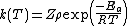.

where:
• Z is the collision frequency
Collision frequency
Collision frequency is defined in chemical kinetics, in the background of theoretical kinetics, as the average number of collisions between reacting molecules per unit of time...

.
•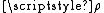is the steric factor
Steric factor
Steric factor, P is a term used in collision theory.It is defined as the ratio between the experimental value of the rate constant and the one predicted by collision theory. It can also be defined as the ratio between the preexponential factor and the collision frequency, and it is most often...

.
• Ea is the activation energy
Activation energy
In chemistry, activation energy is a term introduced in 1889 by the Swedish scientist Svante Arrhenius that is defined as the energy that must be overcome in order for a chemical reaction to occur. Activation energy may also be defined as the minimum energy required to start a chemical reaction...

of the reaction.
• T is the temperature
Temperature
Temperature is a physical property of matter that quantitatively expresses the common notions of hot and cold. Objects of low temperature are cold, while various degrees of higher temperatures are referred to as warm or hot...

.
• R is gas constant
Gas constant
The gas constant is a physical constant which is featured in many fundamental equations in the physical sciences, such as the ideal gas law and the Nernst equation. It is equivalent to the Boltzmann constant, but expressed in units of energy The gas constant (also known as the molar, universal,...

.

The collision frequency is: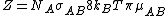where:
• NA is the Avogadro constant
• σAB is the reaction cross section
Cross section (physics)
A cross section is the effective area which governs the probability of some scattering or absorption event. Together with particle density and path length, it can be used to predict the total scattering probability via the Beer-Lambert law....

• kB is Boltzmann's constant
• μAB is the reduced mass
Reduced mass
Reduced mass is the "effective" inertial mass appearing in the two-body problem of Newtonian mechanics. This is a quantity with the unit of mass, which allows the two-body problem to be solved as if it were a one-body problem. Note however that the mass determining the gravitational force is not...

of the reactants.

### Derivation

Consider the reaction:
A + B → C

In collision theory it is considered that two particles A and B will collide if their nuclei get closer than a certain distance. The area around a molecule A in which it can collide with an approaching B molecule is called the cross section (σAB) of the reaction and is, in principle, the area corresponding to a circle whose radius (rAB) is the sum of the radii of both reacting molecules, which are supposed to be spherical.
A moving molecule will therefore sweep a volume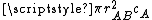per second as it moves, where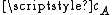is the average velocity of the particle.

From kinetic theory
Kinetic theory
The kinetic theory of gases describes a gas as a large number of small particles , all of which are in constant, random motion. The rapidly moving particles constantly collide with each other and with the walls of the container...

it is known that a molecule of A has an average velocity (different from root mean square
Root mean square
In mathematics, the root mean square , also known as the quadratic mean, is a statistical measure of the magnitude of a varying quantity. It is especially useful when variates are positive and negative, e.g., sinusoids...

velocity) of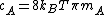, where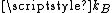is Boltzmann constant and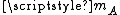is the mass of the molecule.

The solution of the two body problem states that two different moving bodies can be treated as one body which has the reduced mass
Reduced mass
Reduced mass is the "effective" inertial mass appearing in the two-body problem of Newtonian mechanics. This is a quantity with the unit of mass, which allows the two-body problem to be solved as if it were a one-body problem. Note however that the mass determining the gravitational force is not...

of both and moves with the velocity of the center of mass
Center of mass
In physics, the center of mass or barycenter of a system is the average location of all of its mass. In the case of a rigid body, the position of the center of mass is fixed in relation to the body...

, so, in this system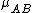must be used instead of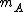.

Therefore, the total collision frequency, of all A molecules, with all B molecules, is: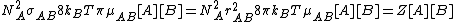From Maxwell Boltzmann distribution it can be deduced that the fraction of collisions with more energy than the activation energy is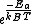. Therefore the rate of a bimolecular reaction for ideal gases will be: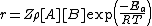Where:
• Z is the collision frequency.
•is the steric factor
Steric factor
Steric factor, P is a term used in collision theory.It is defined as the ratio between the experimental value of the rate constant and the one predicted by collision theory. It can also be defined as the ratio between the preexponential factor and the collision frequency, and it is most often...

, which will be discussed in detail in the next section.
• Ea is the activation energy
Activation energy
In chemistry, activation energy is a term introduced in 1889 by the Swedish scientist Svante Arrhenius that is defined as the energy that must be overcome in order for a chemical reaction to occur. Activation energy may also be defined as the minimum energy required to start a chemical reaction...

of the reaction.
• T is the absolute temperature.
• R is gas constant
Gas constant
The gas constant is a physical constant which is featured in many fundamental equations in the physical sciences, such as the ideal gas law and the Nernst equation. It is equivalent to the Boltzmann constant, but expressed in units of energy The gas constant (also known as the molar, universal,...

.

The product Zρ is equivalent to the preexponential factor of the Arrhenius equation
Arrhenius equation
The Arrhenius equation is a simple, but remarkably accurate, formula for the temperature dependence of the reaction rate constant, and therefore, rate of a chemical reaction. The equation was first proposed by the Dutch chemist J. H. van 't Hoff in 1884; five years later in 1889, the Swedish...

.

### Validity of the theory and steric factor

Once a theory is formulated, its validity must be tested, that is, compare its predictions with the results of the experiments.

When the expression form of the rate constant is compared with the rate equation
Rate equation
The rate law or rate equation for a chemical reaction is an equation that links the reaction rate with concentrations or pressures of reactants and constant parameters . To determine the rate equation for a particular system one combines the reaction rate with a mass balance for the system...

for an elementary bimolecular reaction,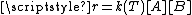, it is noticed that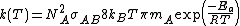.

That expression is similar to the Arrhenius equation
Arrhenius equation
The Arrhenius equation is a simple, but remarkably accurate, formula for the temperature dependence of the reaction rate constant, and therefore, rate of a chemical reaction. The equation was first proposed by the Dutch chemist J. H. van 't Hoff in 1884; five years later in 1889, the Swedish...

, and gives the first theoretical explanation for the Arrhenius equation on a molecular basis. The weak temperature dependence of the preexponential factor is so small compared to the exponential factor that it cannot be measured experimentally, that is, "it is not feasible to establish, on the basis of temperature studies of the rate constant, whether the predicted T½ dependence of the preexponential factor is observed experimentally"

#### Steric factor

If the values of the predicted rate constants are compared with the values of known rate constants it is noticed that collision theory fails to estimate the constants correctly and the more complex the molecules are, the more it fails. The reason for this is that particles have been supposed to be spherical and able to react in all directions; that is not true, as the orientation of the collisions is not always the right one. For example in the hydrogenation
Hydrogenation
Hydrogenation, to treat with hydrogen, also a form of chemical reduction, is a chemical reaction between molecular hydrogen and another compound or element, usually in the presence of a catalyst. The process is commonly employed to reduce or saturate organic compounds. Hydrogenation typically...

reaction of ethylene
Ethylene
Ethylene is a gaseous organic compound with the formula . It is the simplest alkene . Because it contains a carbon-carbon double bond, ethylene is classified as an unsaturated hydrocarbon. Ethylene is widely used in industry and is also a plant hormone...

the H2 molecule must approach the bonding zone between the atoms, and only a few of all the possible collisions fulfill this requirement.

To alleviate this problem, a new concept must be introduced: the steric factor, ρ. It is defined as the ratio between the experimental value and the predicted one (or the ratio between the frequency factor and the collision frequency, and it is most often less than unity.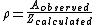Usually, the more complex the reactant molecules, the lower the steric factor. Nevertheless, some reactions exhibit steric factors greater than unity: the harpoon reaction
Harpoon reaction
Harpoon reactions are a type of chemical reaction between two substances one of them prone to form a cation, generally a metal, and the other one prone to form an anion, generally a halogen....

s, which involve atoms that exchange electron
Electron
The electron is a subatomic particle with a negative elementary electric charge. It has no known components or substructure; in other words, it is generally thought to be an elementary particle. An electron has a mass that is approximately 1/1836 that of the proton...

s, producing ion
Ion
An ion is an atom or molecule in which the total number of electrons is not equal to the total number of protons, giving it a net positive or negative electrical charge. The name was given by physicist Michael Faraday for the substances that allow a current to pass between electrodes in a...

s. The deviation from unity can have different causes: the molecules are not spherical, so different geometries are possible; not all the kinetic energy is delivered into the right spot; the presence of a solvent (when applied to solutions), etc.
Experimental rate constants compared to the ones predicted by collision theory for gas phase reactions
Reaction A (Azra frequency factor) Z (collision frequency
Collision frequency
Collision frequency is defined in chemical kinetics, in the background of theoretical kinetics, as the average number of collisions between reacting molecules per unit of time...

)
Steric factor
2ClNO → 2Cl + 2NO 9.4 109 5.9 1010 0.16
2ClO → Cl2 + O2 6.3 107 2.5 1010 2.3 10−3
H2 + C2H4 → C2H6 1.24 106 7.3 1011 1.7 10−6
Br2 + K → KBr + Br 1012 2.1 1011 4.3

Collision theory can be applied to reactions in solution; in that case, the solvent cage has an effect on the reactant molecules and several collisions can take place in a single encounter, which leads to predicted preexponential factors being too large. ρ values greater than unity can be attributed to favorable entropic
Entropy
Entropy is a thermodynamic property that can be used to determine the energy available for useful work in a thermodynamic process, such as in energy conversion devices, engines, or machines. Such devices can only be driven by convertible energy, and have a theoretical maximum efficiency when...

contributions.
Experimental rate constants compared to the ones predicted by collision theory for reactions in solution
Reaction Solvent A 10−11 Z
Collision frequency
Collision frequency is defined in chemical kinetics, in the background of theoretical kinetics, as the average number of collisions between reacting molecules per unit of time...

10−11
Steric factor
C2H5Br
Bromoethane
Bromoethane, also known as ethyl bromide, is a chemical compound of the haloalkanes group. It is abbreviated by chemists as EtBr. This volatile compound has an ether-like odour.-Preparation:...

+ OH-
C2H5OH
Ethanol
Ethanol, also called ethyl alcohol, pure alcohol, grain alcohol, or drinking alcohol, is a volatile, flammable, colorless liquid. It is a psychoactive drug and one of the oldest recreational drugs. Best known as the type of alcohol found in alcoholic beverages, it is also used in thermometers, as a...

4.30 3.86 1.11
C2H5O-
Ethanol
Ethanol, also called ethyl alcohol, pure alcohol, grain alcohol, or drinking alcohol, is a volatile, flammable, colorless liquid. It is a psychoactive drug and one of the oldest recreational drugs. Best known as the type of alcohol found in alcoholic beverages, it is also used in thermometers, as a...

+ CH3I
Iodomethane
Methyl iodide, also called iodomethane, and commonly abbreviated "MeI", is the chemical compound with the formula CH3I. It is a dense, colorless, volatile liquid. In terms of chemical structure, it is related to methane by replacement of one hydrogen atom by an atom of iodine. It is naturally...

C2H5OH 2.42 1.93 1.25
ClCH2CO2- + OH- water
Water
Water is a chemical substance with the chemical formula H2O. A water molecule contains one oxygen and two hydrogen atoms connected by covalent bonds. Water is a liquid at ambient conditions, but it often co-exists on Earth with its solid state, ice, and gaseous state . Water also exists in a...

4.55 2.86 1.59
C3H6Br2 + I- CH3OH
Methanol
Methanol, also known as methyl alcohol, wood alcohol, wood naphtha or wood spirits, is a chemical with the formula CH3OH . It is the simplest alcohol, and is a light, volatile, colorless, flammable liquid with a distinctive odor very similar to, but slightly sweeter than, ethanol...

1.07 1.39 0.77
HOCH2CH2Cl + OH- water 25.5 2.78 9.17
4-CH3C6H4O-
Cresol
Cresols are organic compounds which are methylphenols. They are a widely occurring natural and manufactured group of aromatic organic compounds which are categorized as phenols . Depending on the temperature, cresols can be solid or liquid because they have melting points not far from room...

+ CH3I
ethanol 8.49 1.99 4.27
CH3(CH2)2Cl + I- (CH3)2CO
Acetone
Acetone is the organic compound with the formula 2CO, a colorless, mobile, flammable liquid, the simplest example of the ketones.Acetone is miscible with water and serves as an important solvent in its own right, typically as the solvent of choice for cleaning purposes in the laboratory...

0.085 1.57 0.054
C5H5N
Pyridine
Pyridine is a basic heterocyclic organic compound with the chemical formula C5H5N. It is structurally related to benzene, with one C-H group replaced by a nitrogen atom...

+ CH3I
C2H2Cl4 - - 2.0 10−6